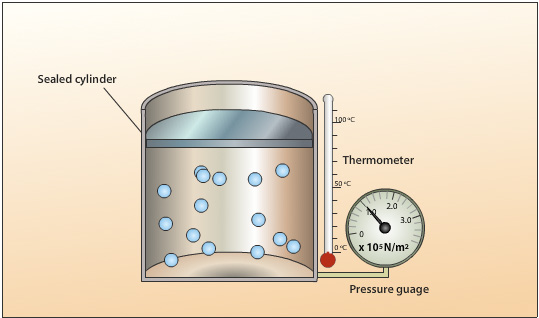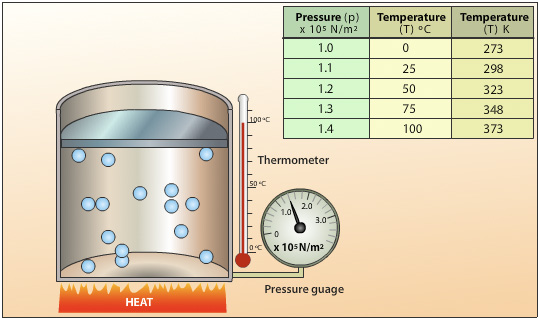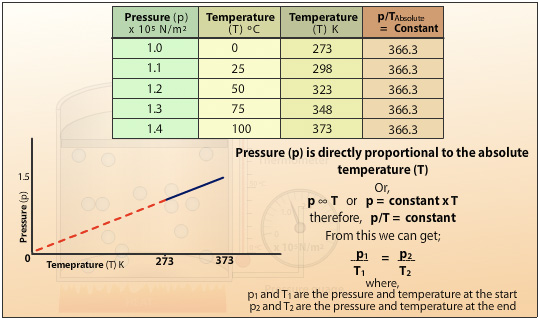## The Pressure Law

The pressure law states:

"For a fixed mass of gas, at a constant volume, the pressure (p) is directly proportional to the absolute temperature (T)."

Pressure Temperature

 Pressure = constant Temperature

The animation below gives and explanation of the Pressure law:A sealed cylinder with no leaks contains a fixed mass. The volume of the gas is kept constant by using a cylinder with a fixed roof capable of withstanding high pressures.The gas pressure is created by the collision of the moving gas particles with each other and against the walls of the cylinder.The following set up is used to investigate the relationship between temperature and pressure for a gas. Heat energy is applied to the cylinder and the temperature of the gas increases. The average velocity of the gas particles increases resulting in an increase in the rate of collisions and the average force per collision. Because the areas of the walls are kept constant, the force per unit area increases resulting in an increase in pressure.Plotting the pressure (p) against the absolute temperature (T) gives a straight line which when extrapolated passes through the origin. This shows the pressure of the gas is directly proportional to the absolute temperature of the gas. Doubling the temperature will double the pressure for a fixed mass of gas at constant volume. The gradient of the slope is the constant in Charles’ Law. It also shows that if the gas is cooled to absolute zero then the energy of the molecules is at the lowest energy state and therefore cannot generate any pressure.

## Pressure Law Example:

Using the example of the sealed cylinder above the pressure of gas is recorded as 1.0 x 105 N/m2 at a temperature of 0°C. The cylinder is heated further till the thermometer records 150°C. What is the pressure of the gas?

### Solution:

We know

p/T = constant

therefore,

p1/T1 = p2/T2

p1 = 1.0 x 105 N/m2
T1 = 0°C = 0+273 = 273K (remember to convert from Celsius to Kelvin)
T2 = 150°C = 150+273 = 423K
p2  =
?

p1/T1 = p2/T2

 p2 = p1x T2 T1

 p2 = 1.0 x 105 x 423 273

= 1.54 x 105 N/m2